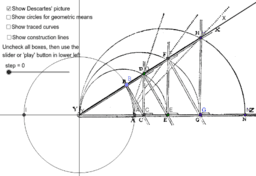•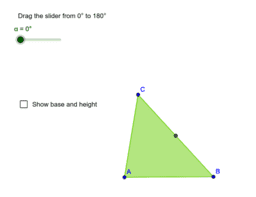The area of a triangle

Activity

•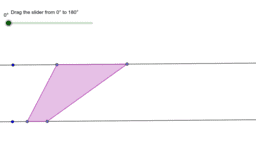Dissecting a Trapezoid to a Rectangle

Activity

•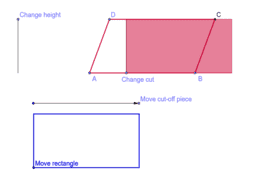Dissecting a parallelogram to a rectangle

Activity

•The focus-directrix construction of an ellipse

Activity

•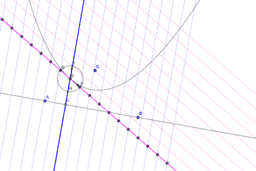Coordinate system for a parabola (Apollonius)

Activity

•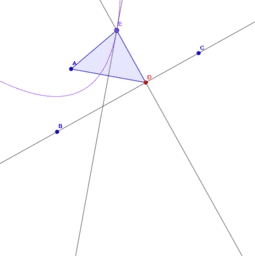The focus-directrix construction of a parabola

Activity

•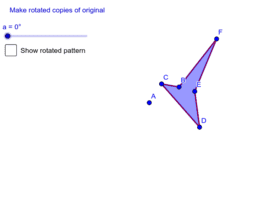Creating a pattern with rotational symmetry

Activity

•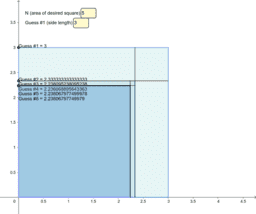An Indian Square Root Algorithm

Activity

•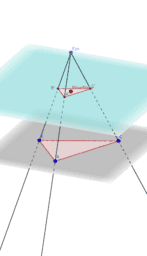Similarity: Dilations and Cones

Activity

•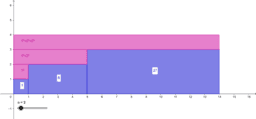Al Hazen's Summation Formulas, Degree 3

Activity

•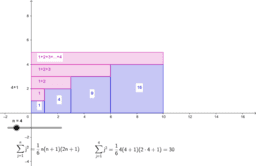Al Hazen's Summation Formulas, Degree 2

Activity

•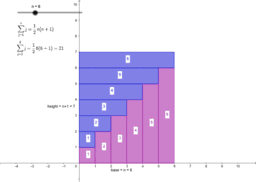Al Hazen's summation formulas, degree 1

Activity

•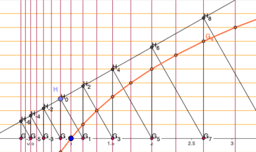Descartes's Logarithm Graph

Activity

•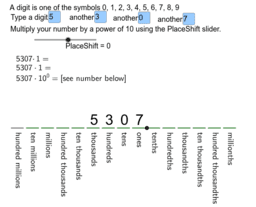Place value and multiplication by powers of ten

Activity

•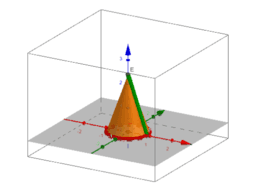Cone from a sector

Activity

•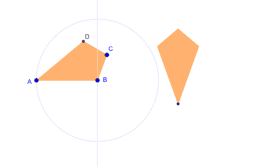Dynamic kite

Activity

•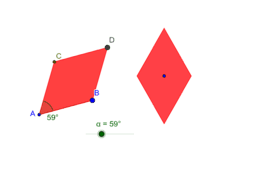Dynamic rhombus

Activity

•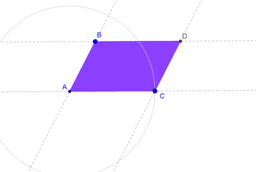Dynamic parallelogram

Activity

•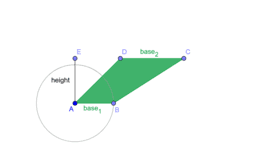Dynamic trapezoid

Activity

•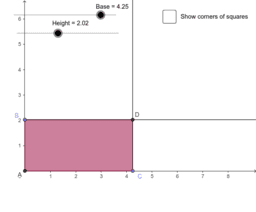Dynamic rectangle

Activity

•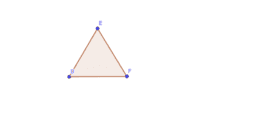Construction #2

Activity

•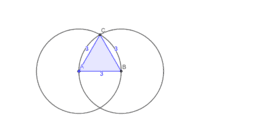Construction #3

Activity

•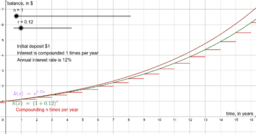Compound interest and exponential functions

Activity

•